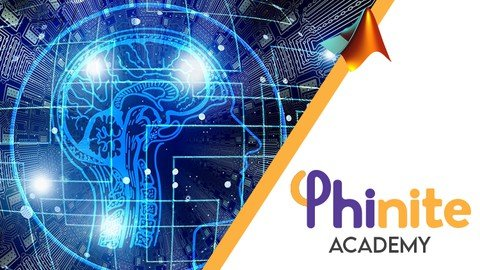﻿ Backpropagation Learning Method in Matlab » GFXhome WS

Information of news
2-07-2021, 23:03

### Backpropagation Learning Method in Matlab

Category: TutorialsBackpropagation Learning Method in Matlab
Genre: eLearning | MP4 | Video: h264, 1280x720 | Audio: AAC, 48.0 KHz
Language: English | Size: 1.31 GB | Duration: 3h 21mLearn to Develop Neural Network Methods in Matlab without using any Tools.

==========

What you'll learn
You will learn the basics of Artificial Intelligence and Machine Learning very solidly.
You will learn important Machine Learning algorithms.
You will learn The Backpropagation Algorithm deeply using only basic Math!

Description
Optimization techniques are used in the solution of many engineering problems today. Especially in cases where modeling cannot be done, solutions are achieved by applying iterative methods with the help of mathematical equations. These applications in linear or non-linear equation sets are now performed everywhere in parallel to the development of computer architectures.

Artificial neural networks, one of the mathematical problems that can be solved using optimization techniques, are frequently applied to find the solution of data/equation sets that cannot be modeled. These structures, which can establish a relationship between mathematically given output/input signals, are actively utilized in the imitation or classification of any signal. On the other hand, the solution for the given input/output data cannot be found analytically, unfortunately. Therefore, it requires the use of optimization techniques derived by iterative methods.

In order for artificial neural networks to reach a solution, a rule inference that minimizes a defined cost function is required. The name of this rule is given by learning methods. The backpropagation learning method is obtained by using the first-order derivative rule in order to minimize the error. Therefore, the extraction and application of this rule are necessary and essential for training an artificial neural network model.

Writing a code to mathematical equations and iterative solvers in software languages ​​is one of the biggest problems of students who want to build their own software program since it is necessary to use complex equation sets with loop/condition expressions and to eliminate the effects of disturbing situations such as bug, etc. Therefore, the writing of the mathematically obtained equations with simple expressions in software languages ​​is very important for developers or engineering students.

For all these reasons, the mathematical basis of the backpropagation learning method and its application in software languages ​​are covered in this course. First of all, it is explained mathematically what artificial neural networks are. After that, it is shown how to minimize a cost function by using advanced mathematical calculation. Then, it is shown how the backpropagation learning method can be obtained for an artificial neural network model in the programming language.

How to write these inferred complex equations as functions in MATLAB programming language is explained in this course. The writing of a complex library, how to write a seamless learning method, and how to obtain other rules with these methods are given in detail in this course.

Backpropagation Learning Method in Matlab

or
https://rapidgator.net/file/ed3a1db5647ed771b75cf26517af5c8d/Backpropagation_Learning_Method_in_Matlab.part1.rar.html
https://rapidgator.net/file/55b76edce7d6e079b696eacfa10f2e68/Backpropagation_Learning_Method_in_Matlab.part2.rar.html[/QUOTE]Site BBcode/HTML Code:
Dear visitor, you went to the site as unregistered user.